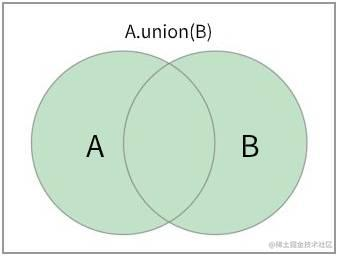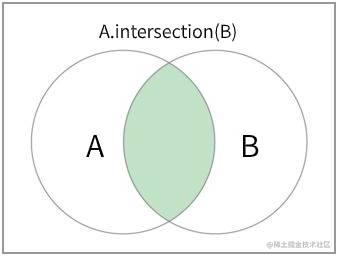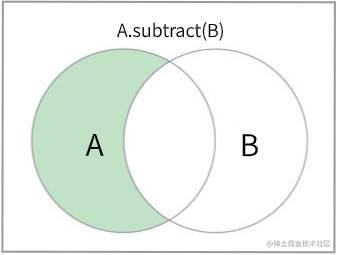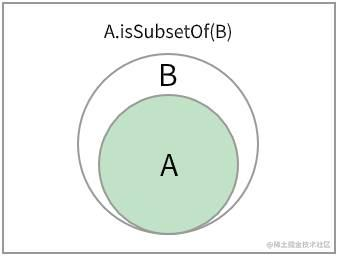## 实现集合

### 定义集合类

``````class Set {
constructor() {
this.items = {}
}
}

• delete(element) 从集合中移除一个元素
• has(element) 判断一个元素是否在集合中
• clear() 移除集合中的所有元素
• size() 返回集合中所包含元素的数量
• values() 返回一个包含集合中所有元素的数组
• isEmpty() 判断集合是否为空
• size() 返回集合中元素个数
• clear() 清空集合
• toString() 将集合中的元素以字符串形式输出

``````add(element) {
if (!this.has(element)) {
this.items[element] = element;
return true;
}

return false;
}

### delete 从集合中移除一个元素

``````delete(element) {
if (this.has(element)) {
delete this.items[element];
return true;
}

return false;
}

### has 判断一个元素是否在集合中

``````has(element) {
return Object.prototype.hasOwnProperty.call(this.items, element);
}

### clear 移除集合中的所有元素

``````clear() {
this.items = {}
}

### size 返回集合中所包含元素的数量

``````size() {
return Object.keys(this.items).length;
}

``````size() {
let count = 0;
for (let key in this.items) {
if (this.items.hasOwnProperty(key)) {
count++
}
}
return count;
}

### values 返回一个包含集合中所有元素的数组

``````values() {
return Object.values(this.items);
}

``````values() {
let values = [];
for (let key in this.items) {
if (this.items.hasOwnProperty(key)) {
// 注意，我们保存的集合元素 key 与 value 相同，因此可以直接将 key  push 进 values 中
values.push(key)
}
}
}

### isEmpty 判断集合是否为空

``````isEmpty() {
return this.size() === 0;
}

### toString 将集合中的元素以字符串形式输出

``````toString() {
if (this.isEmpty()) {
return '';
}

const values = this.values();
let objString = `\${values}`;
for (let i = 1; i < values.length; i++) {
objString = `\${objString}, \${values[i].toString()}`;
}

return objString;
}

## 集合运算

• 并集：对于给定的两个集合，返回一个包含两个集合中所有元素的新集合
• 交集：对于给定的两个集合，返回一个包含两个集合中共有元素的集合
• 差集：对于给定的两个集合，返回一个包含所有存在于第一个集合且不存在于第二个集合的元素的新集合
• 子集：验证一个给定集合是否是另一个集合的子集

### 并集

`A ∪ B`

`A ∪ B = {x | x ∈ A ∨ x ∈ B}```````union(otherSet) {
// 存放并集的结果
const unionSet = new Set();
// 迭代当前集合的所有值，将值添加到并集 集合中
// 迭代 otherSet 的所有值，将值添加到并集 集合中
return unionSet;
}

### 交集

`A ∩ B`

`A ∩ B = {x | x ∈ A ∧ x ∈ B}```````intersection(otherSet) {
// 存放交集的结果
const intersectionSet = new Set();
// 当前集合（当前 Set 类的实例）的所有值
const values = this.values();
// 第二个集合的所有值
const otherValues = otherSet.values();

// 假设当前的集合元素较多
let biggerSet = values;
// 假设第二个集合元素较少
let smallerSet = otherValues;

// 比较两个集合的元素个数，如果另外一个集合的元素多于当前集合，则交换 biggerSet 和 smallerSet
if (otherValues.length - values.length > 0) {
biggerSet = otherValues;
smallerSet = values;
}

// 迭代较小的集合，计算出两个集合共有元素添加到 交集 集合中
smallerSet.forEach(value => {
if (biggerSet.includes(value)) {
}
})

// 将交集结果返回
return intersectionSet;
}

intersection 方法会得到所有同时存在于两个集合中的元素。我们首先创建一个新的集合 intersectionSet 来存放交集的结果。然后分别获取当前 Set 实例中的所有元素和另一个集合中的所有元素。我们假定当前集合的元素较多，另一个集合的元素较少，比较两个集合的元素个数，如果另一个集合的元素个数多于当前集合中的元素个数，我们就交换 biggerSet 和 smallerSet 的值。最后迭代较小集合 smallerSet 来计算出两个集合的共有元素。

### 差集

`A﹣B`

`A﹣B = {x | x ∈ A ∧ x ∉ B}```````subtract(otherSet) {
// 存放差集结果
const subtractionSet = new Set();
// 迭代当前集合的所有值
this.values().forEach(value => {
// 当前值不存在于 otherSet 中，将其添加到 差集 集合中
if (!otherSet.has(value)) {
// 将元素添加到集合中
}
})

return subtractionSet;
}

subtract 方法会得到所有存在于集合 A 但不存在于集合 B 的元素。我们首先创建一个新的集合变量 subtractionSet 来存放差集结果。通过 this.values() 拿到集合A 中的所有值，然后迭代集合 A 中的所有值，将存在于集合A 但不存在于集合B 中的元素放入 subtractionSet 集合中，迭代完集合 A 中的所有元素后，subtractionSet 集合中的元素就是集合A 与集合B 差集。

### 子集

`A ⊆ B`

`{x|∀x∈A ⇒ x ∈ B}```````isSubsetOf(otherSet) {
// 验证当前 Set 实例的大小
// 当前 Set 实例的元素多于 otherSet，那么当前 Set 实例不是 otherSet 的子集
if (this.size() > otherSet.size()) {
return false;
}

// 假定当前 Set 实例是给定集合的子集
let isSubset = true;
// 迭代当前 Set 实例的所有元素
this.values().every(value => {
// 有任何元素不存在于 otherSet 中，那么当前 Set 实例就不是 otherSet 的子集
if (!otherSet.has(value)) {
isSubset = false;
return false;
}
return true;
})
return isSubset;
}

isSubsetOf 方法验证 集合 A 是否是 集合 B 的子集。我们首先需要验证当前 Set 实例的大小（集合A），如果当前实例中的元素比 otherSet 实例（集合B）更多，说明当前 Set 实例不是 otherSet 的子集。（子集的元素个数需要小于等于要比较的集合）。

## 完整代码

``````class Set {
constructor() {
this.items = {};
}

// 向集合添加一个新元素
if (!this.has(element)) {
this.items[element] = element;
return true;
}

return false;
}

// 从集合中移除一个元素
delete(element) {
if (this.has(element)) {
delete this.items[element];
return true;
}

return false;
}

// 判断一个元素是否存在于集合中
has(element) {
return Object.prototype.hasOwnProperty.call(this.items, element);
}

// 移除集合中的所有元素
clear() {
this.items = {};
}

// 返回集合中所包含元素的数量
size() {
let count = 0;
for(let key in this.items) {
if (this.items.hasOwnProperty(key)) {
count++;
}
}

return count;
}

// 返回一个包含集合中所有元素的数组
values() {
let values = [];
for (let key in this.items) {
if (this.items.hasOwnProperty(key)) {
values.push(key);
}
}
return values;
}

// 并集
union(otherSet) {
const unionSet = new Set();
return unionSet;
}

// 交集
intersection(otherSet) {
const intersectionSet = new Set();
const values = this.values();
const otherValues = otherSet.values();

let biggerSet = values;
let smallerSet = otherValues;
if (otherValues.length - values.length > 0) {
biggerSet = otherValues;
smallerSet = values;
}

smallerSet.forEach(value => {
if (biggerSet.includes(value)) {
}
})

return intersectionSet;
}

// 差集
subtract(otherSet) {
const subtractionSet = new Set();
this.values().forEach(value => {
if (!otherSet.has(value)) {
}
})

return subtractionSet;
}

// 子集
isSubsetOf(otherSet) {
if (this.size() > otherSet.size()) {
return false;
}

let isSubset = true;
this.values().every(value => {
if (!otherSet.has(value)) {
isSubset = false;
return false;
}
return true;
})
return isSubset;
}

}

## ES6 Set 类的集合运算

### 并集

``````// A 和 B 的并集
function union(setA, setB) {
const unionSet = new Set([...setA, ...setB]);
return unionSet;
}

### 交集

``````// A 和 B 的交集
function intersection(setA, setB) {
const intersect = new Set([...a].filter(x => b.has(x)));
return intersect
}

### 差集

``````// A 相对于 B 的差集
function subTract(setA, setB) {
const subTractSet = new Set([...a].filter(x => !b.has(x)));
return subTractSet;
}479